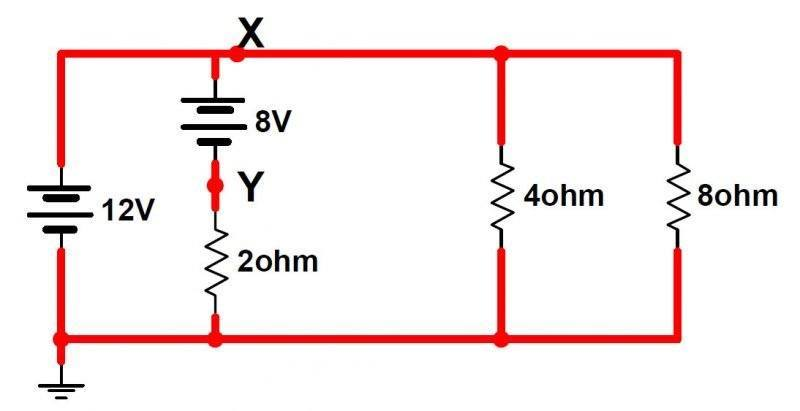# Kirchhoff's and Ohm's law circuit diagram issue.

• Benthal

## Homework Statement

The question requires viewing the circuit diagram to start. An image is attached, it can also be viewed here (http://imgur.com/6Q0DCQ9).The questions are:

a) Calculate the equivalent resistance ##R_T## of the 4 ##\parallel## 8 ohm combination.

b) Redraw the circuit with ##R_T## replacing the two parallel resistors.

c) What is the voltage at point X ?

## Homework Equations

Ohm's law ## V = I R ## , Resistance in parallel ## 1/R_T = 1/R_1 + 1/R_2 ## ,

## The Attempt at a Solution

a)
## 1/R_T = 1/4 + 1/8 ##
## R_T = 2.7 ## Ohm[/B]

b) I just re drew the circuit diagram the same, but instead of the two parallel resistors there is just one with a resistance of 2.7 Ohms.

c) This is where I am stuck. I can't work out how this circuit operates.

I take it the two batteries can't be in parallel because they are different voltages?

So will the current flow back through the 8 v battery and the voltage will drop to 4v at point Y ? And then the voltage stay at 12v at point X ? However now if I calculate the current at the resistors it's different.

I will be extremely grateful if someone can point me in the right direction on this.

Hi Benthal, Welcome to Physics Forums.

Presumably they're looking for the potential at node X with respect to the reference node represented by the ground symbol. See if you can't find a path from the ground point to the X node where you can sum up known potential changes along the way (a "KVL Walk") ;)

Hi gneill,

Thanks for the reply. I've attached a circuit diagram I've drawn with arrows showing the path of the current. Is this the correct path of the current? If so, then I would say there is a potential of 12v between the ground and node X.

#### Attachments

Hi gneill,

Thanks for the reply. I've attached a circuit diagram I've drawn with arrows showing the path of the current. Is this the correct path of the current? If so, then I would say there is a potential of 12v between the ground and node X.
Your current labels are fine. But really you only need to find one path from the reference node to the point of interest for which you want to know the relative potential along which you can sum known potential changes. What path did you follow?

Yup, that's great. Well done.

Excellent, thanks for the help, I've figured it out now. I wasn't thinking about Kirchoff's Current law.